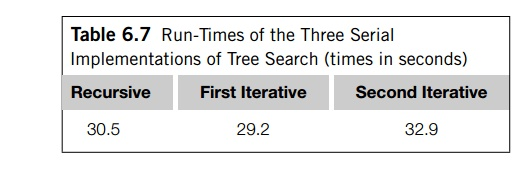Home | | Multi - Core Architectures and Programming | Evaluating the Pthreads tree-search programs

# Evaluating the Pthreads tree-search programs

Table 6.8 shows the performance of the two Pthreads programs on two fifteen-city problems. The “Serial” column gives the run-time of the second iterative solution— the solution that pushes a copy of each new tour onto the stack.

Table 6.8 shows the performance of the two Pthreads programs on two fifteen-city problems. The “Serial” column gives the run-time of the second iterative solution— the solution that pushes a copy of each new tour onto the stack. For reference, the first problem in Table 6.8 is the same as the problem the three serial solutions were tested with in Table 6.7, and both the Pthreads and serial implementations were tested on the same system. Run-times are in seconds, and the numbers in parentheses next to the run-times of the program that uses dynamic partitioning give the total number of times the stacks were split.From these numbers, it’s apparent that different problems can result in radically different behaviors. For example, the program that uses static partitioning generally performs better on the first problem than the program that uses dynamic partitioning. However, on the second problem, the performance of the static program is essentially independent of the number of threads, while the dynamic program obtains excellent performance. In general, it appears that the dynamic program is more scalable than the static program.

As we increase the number of threads, we would expect that the size of the local stacks will decrease, and hence threads will run out of work more often. When threads are waiting, other threads will split their stacks, so as the number of threads is increased, the total number of stack splits should increase. Both problems confirm this prediction.

It should be noted that if the input problem has more than one possible solution— that is, different tours with the same minimum cost—then the results of both of the programs are nondeterministic. In the static program, the sequence of best tours depends on the speed of the threads, and this sequence determines which tree nodes are examined. In the dynamic program, we also have nondeterminism because dif-ferent runs may result in different places where a thread splits its stack and variation in which thread receives the new work. This can also result in run-times, especially dynamic run-times, that are highly variable.

Study Material, Lecturing Notes, Assignment, Reference, Wiki description explanation, brief detail
An Introduction to Parallel Programming : Parallel Program Development : Evaluating the Pthreads tree-search programs |